1. Which of the following shapes is a rectangle?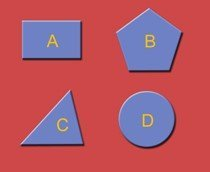2. The angle in the picture below is a(n) …….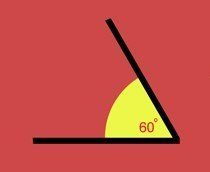3.The angle in the picture below is a(n) …….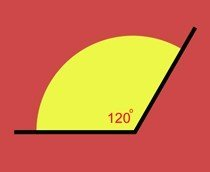4. The angle in the picture below is a(n) …….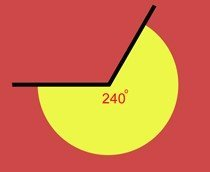5. What is ‘angle A’ in the diagram below?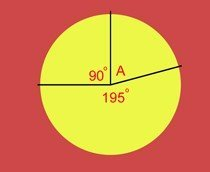Question 1 of 5